Miscellaneous

Chapter 9 Class 11 Sequences and Series (Term 1)
Serial order wise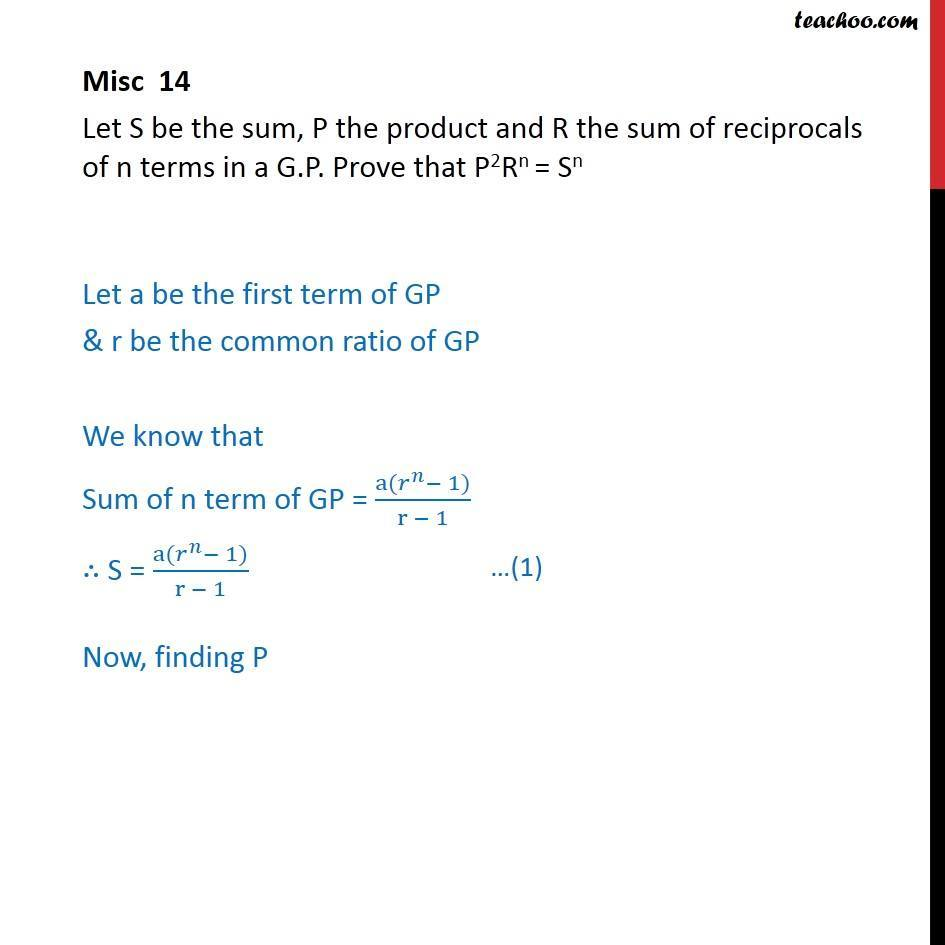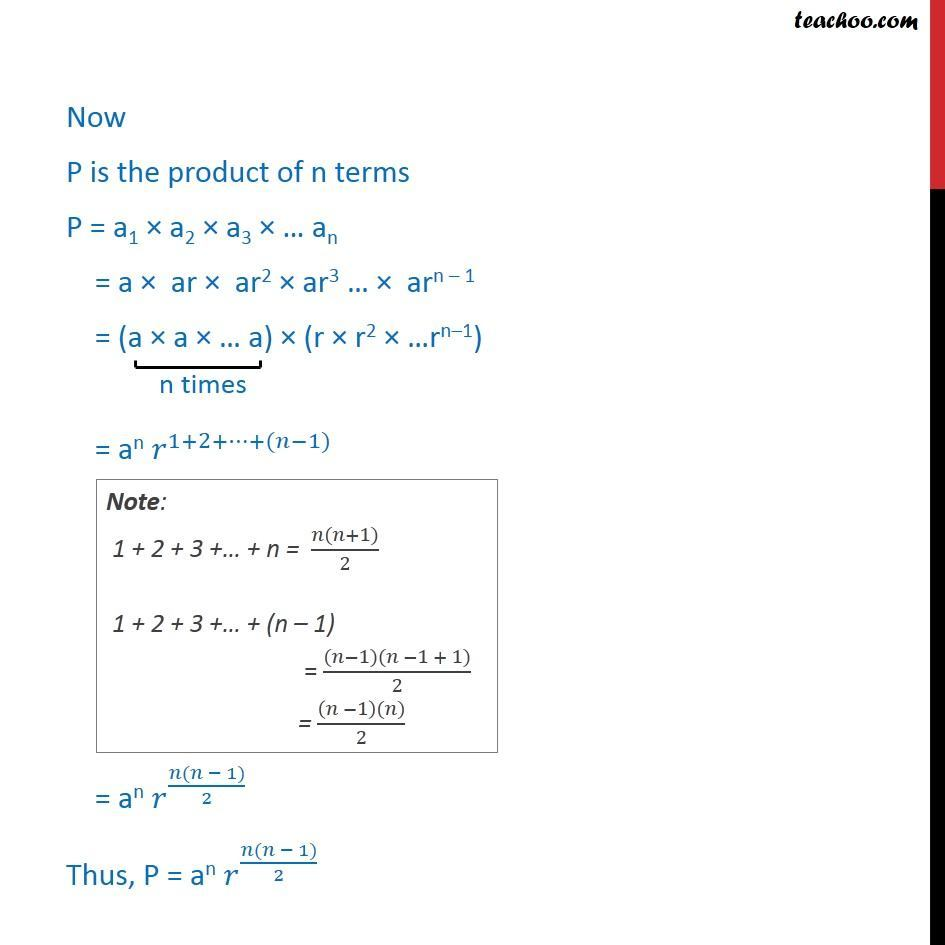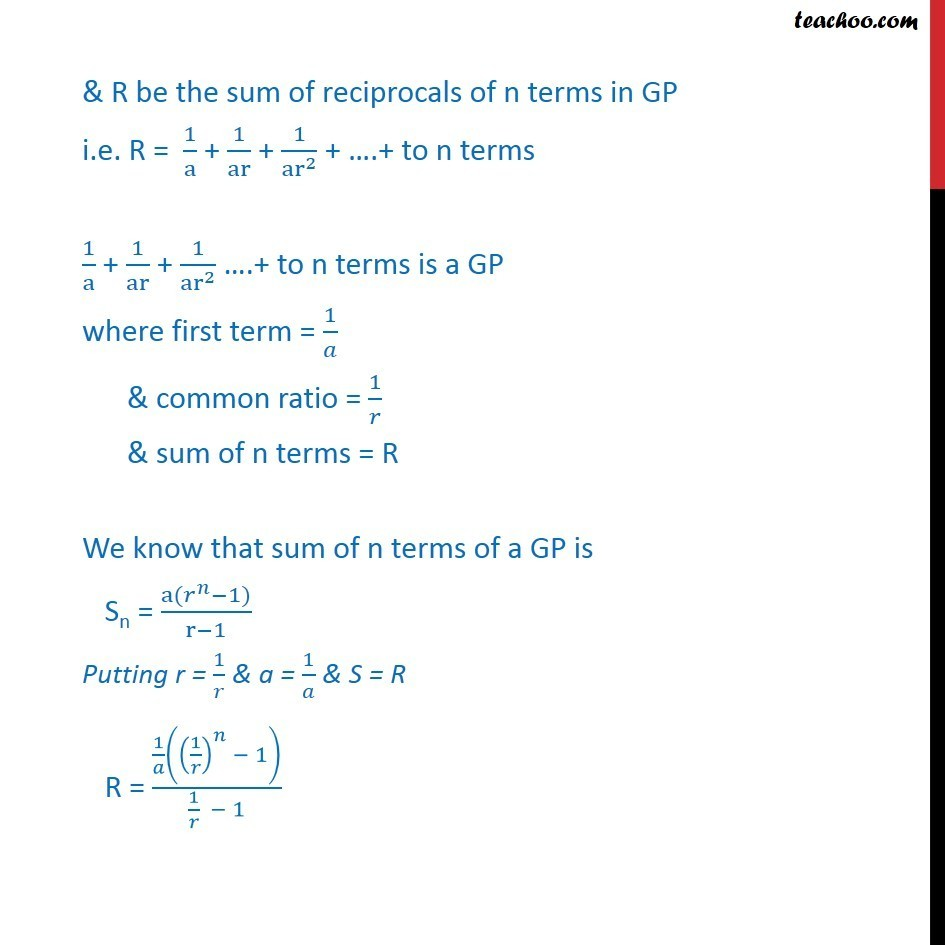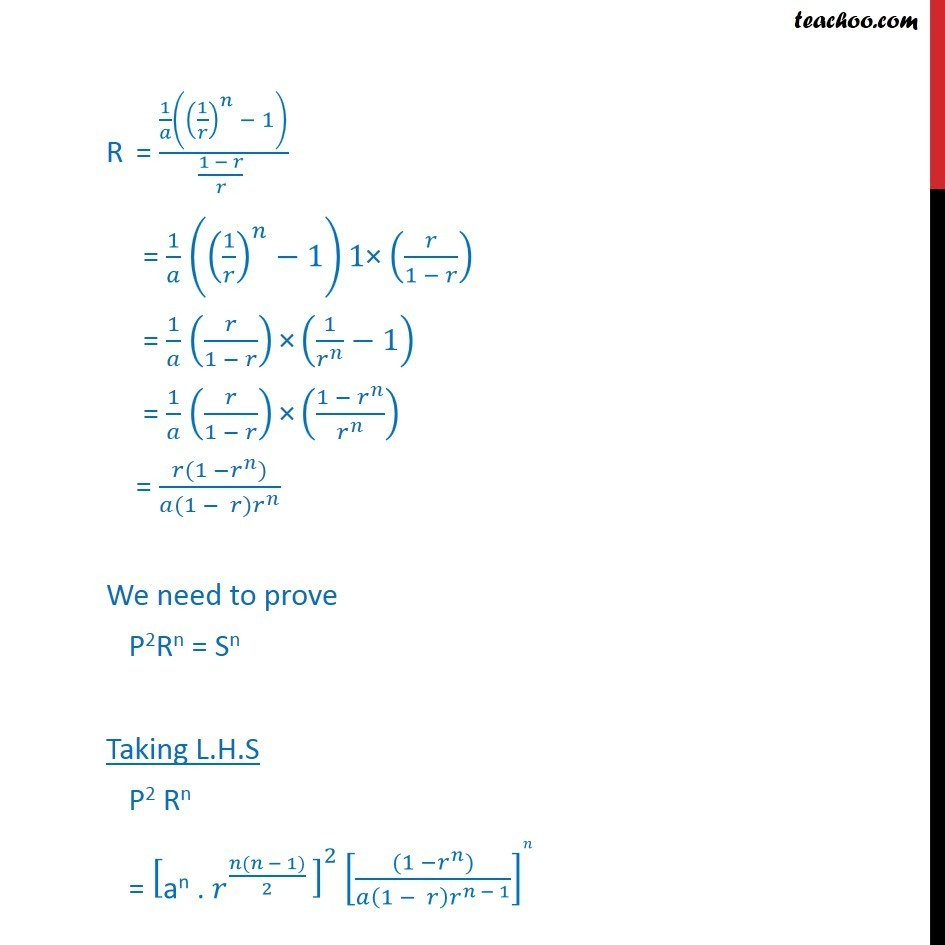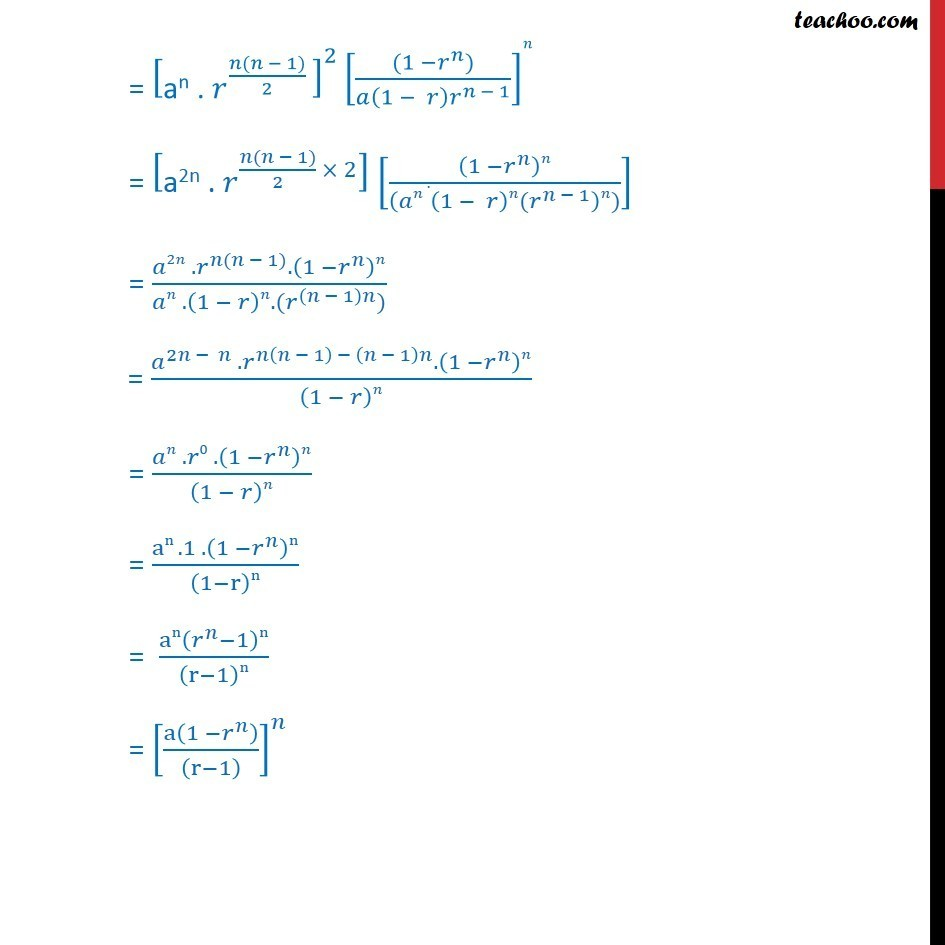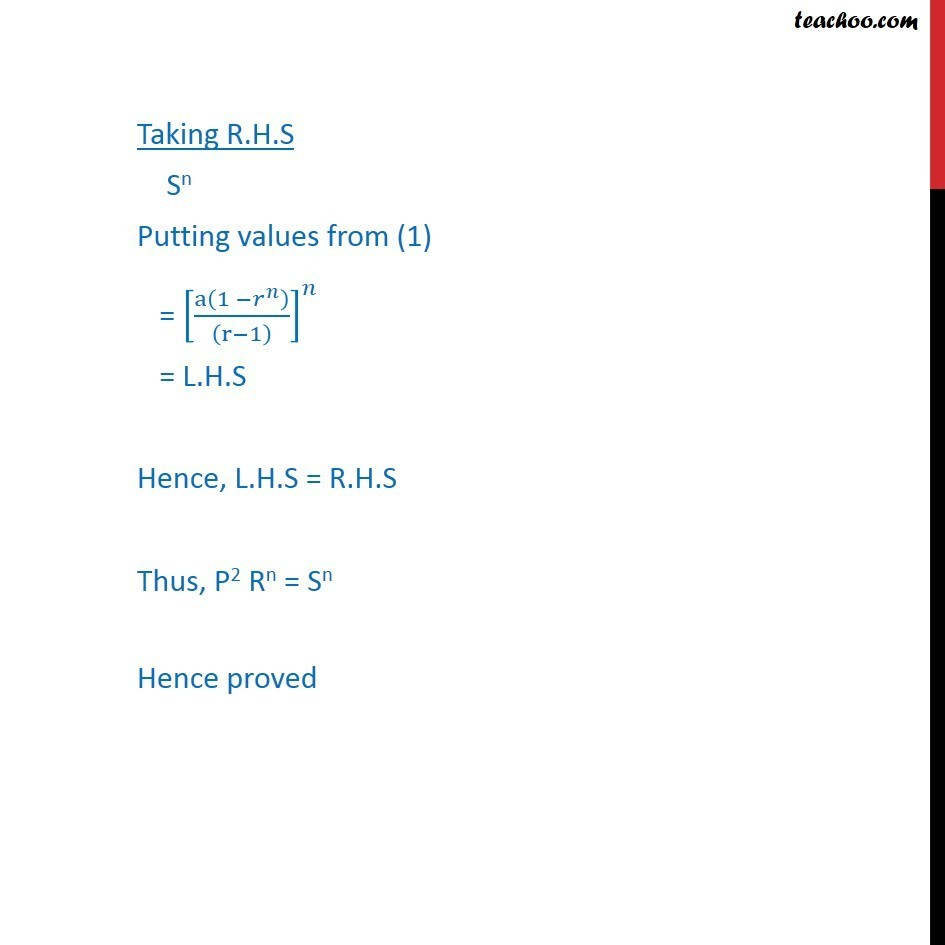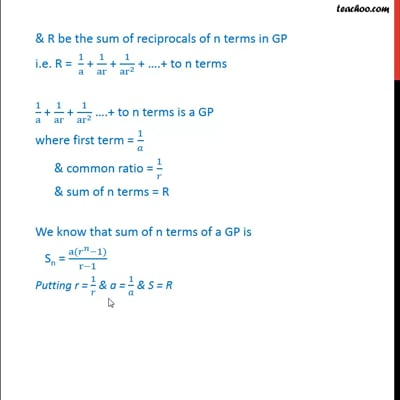This video is only available for Teachoo black users

### Transcript

Misc 14 Let S be the sum, P the product and R the sum of reciprocals of n terms in a G.P. Prove that P2Rn = Sn Let a be the first term of GP & r be the common ratio of GP We know that Sum of n term of GP = (a(𝑟^𝑛− 1))/(r − 1) ∴ S = (a(𝑟^𝑛− 1))/(r − 1) Now, finding P Now P is the product of n terms P = a1 × a2 × a3 × … an = a × ar × ar2 × ar3 … × arn – 1 = (a × a × … a) × (r × r2 × …rn–1) = an 𝑟^(1+2+…+(𝑛−1)) = an 𝑟^((𝑛(𝑛 − 1))/2) Thus, P = an 𝑟^((𝑛(𝑛 − 1))/2) & R be the sum of reciprocals of n terms in GP i.e. R = 1/a + 1/ar + 1/ar^2 + ….+ to n terms 1/a + 1/ar + 1/ar^2 ….+ to n terms is a GP where first term = 1/𝑎 & common ratio = 1/𝑟 & sum of n terms = R We know that sum of n terms of a GP is Sn = (a(𝑟^𝑛−1))/(r−1) Putting r = 1/𝑟 & a = 1/𝑎 & S = R R = (1/𝑎 ((1/𝑟)^𝑛 − 1))/(1/𝑟 − 1) R = (1/𝑎 ((1/𝑟)^𝑛 − 1))/((1 − 𝑟)/𝑟 ) = 1/𝑎 ((1/𝑟)^𝑛−1)1× (𝑟/(1 − 𝑟)) = 1/𝑎 (𝑟/(1 − 𝑟))"×" (1/𝑟^𝑛 −1) = 1/𝑎 (𝑟/(1 − 𝑟))"×" ((1 − 𝑟^𝑛)/𝑟^𝑛 ) = (𝑟(1 −𝑟^𝑛))/(𝑎(1 − 𝑟)𝑟^𝑛 ) We need to prove P2Rn = Sn Taking L.H.S P2 Rn = ["an . " 𝑟^((𝑛(𝑛 − 1))/2 ) ]^2 [((1 −𝑟^𝑛))/(𝑎(1 − 𝑟) 𝑟^(𝑛 − 1) )]^𝑛 = ["an . " 𝑟^((𝑛(𝑛 − 1))/2 ) ]^2 [((1 −𝑟^𝑛))/(𝑎(1 − 𝑟) 𝑟^(𝑛 − 1) )]^𝑛 = ["a2n . " 𝑟^((𝑛(𝑛 − 1))/2 × 2) ] [(1 −𝑟^𝑛 )𝑛/((𝑎𝑛 . (1 − 𝑟)𝑛〖(𝑟〗^(𝑛 − 1) )𝑛))] = (𝑎2𝑛 . 𝑟^(𝑛(𝑛 − 1)). (1 −𝑟^𝑛 )𝑛)/(𝑎𝑛 .(1 − 𝑟)𝑛. (𝑟^(𝑛 − 1)𝑛)) = (𝑎^(2𝑛 − 𝑛) . 𝑟^(𝑛(𝑛 − 1) − (𝑛 − 1)𝑛). (1 −𝑟^𝑛 )𝑛)/(1 − 𝑟)𝑛 = (𝑎𝑛 . 𝑟0 . (1 −𝑟^𝑛 )𝑛)/(1 − 𝑟)𝑛 = (an . 1 .(1 −𝑟^𝑛 )n)/(1−r)n = an(𝑟^𝑛−1)n/(r−1)n = [a(1 −𝑟^𝑛 )/((r−1) )]^𝑛 Taking R.H.S Sn Putting values from (1) = [a(1 −𝑟^𝑛 )/((r−1) )]^𝑛 = L.H.S Hence, L.H.S = R.H.S Thus, P2 Rn = Sn Hence proved